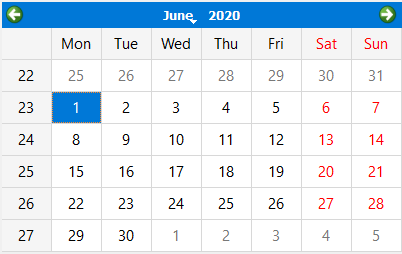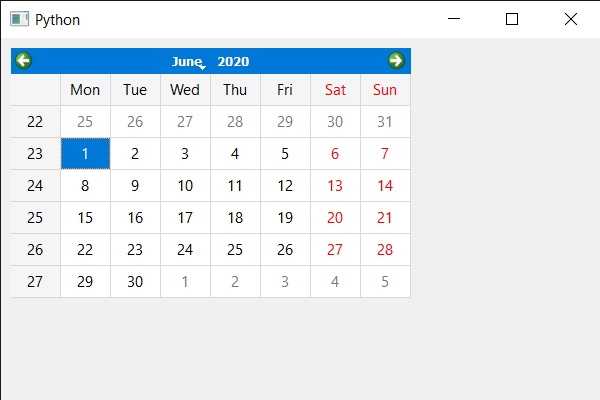Open in App
Not now

# PyQt5 QCalendarWidget – Setting Grid

• Last Updated : 16 Jul, 2021

In this article, we will see how we can set a grid in the QCalendarWidget. Grid is basically the interconnected network of lines that separates the dates from each other. By default, there is no grid to the QCalendarWidget although we can set the grid any time. Below is how a calendar with a grid looks like.In order to do this we will use setGridVisible method with the QCalendarWidget object.
Syntax : calendar.setGridVisible(True)
Argument : It takes bool as argument
Return : It return None

Below is the implementation

## Python3

 `# importing libraries``from` `PyQt5.QtWidgets ``import` `*``from` `PyQt5 ``import` `QtCore, QtGui``from` `PyQt5.QtGui ``import` `*``from` `PyQt5.QtCore ``import` `*``import` `sys`  `class` `Window(QMainWindow):` `    ``def` `__init__(``self``):``        ``super``().__init__()` `        ``# setting title``        ``self``.setWindowTitle(``"Python "``)` `        ``# setting geometry``        ``self``.setGeometry(``100``, ``100``, ``600``, ``400``)` `        ``# calling method``        ``self``.UiComponents()` `        ``# showing all the widgets``        ``self``.show()` `    ``# method for components``    ``def` `UiComponents(``self``):` `        ``# creating a QCalendarWidget object``        ``calendar ``=` `QCalendarWidget(``self``)` `        ``# setting geometry to the calendar``        ``calendar.setGeometry(``10``, ``10``, ``400``, ``250``)` `        ``# setting grid``        ``calendar.setGridVisible(``True``)`  `# create pyqt5 app``App ``=` `QApplication(sys.argv)` `# create the instance of our Window``window ``=` `Window()` `# start the app``sys.exit(App.``exec``())`

Output :My Personal Notes arrow_drop_up